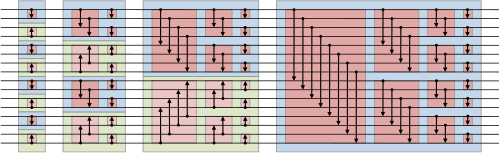# OpenCL Sorting

Eric Bainville - June 2011

## Parallel bitonic, local

Here again, we sort in local memory. We execute N threads in workgroups of WG threads, and each workgroup sorts a segment of WG input records. The output contains N/WG ordered sequences.

Ken Batcher's bitonic sorting network is described in Knuth's book, and nicely illustrated in the Wikipedia page:Bitonic sorter network (from Wikipedia).

The code below is a direct translation of the network pictured in the figure, where each thread computes exactly one element (i.e. we have two threads for each comparator).

```__kernel void ParallelBitonic_Local(__global const data_t * in,__global data_t * out,__local data_t * aux)
{
int i = get_local_id(0); // index in workgroup
int wg = get_local_size(0); // workgroup size = block size, power of 2

// Move IN, OUT to block start
int offset = get_group_id(0) * wg;
in += offset; out += offset;

aux[i] = in[i];
barrier(CLK_LOCAL_MEM_FENCE); // make sure AUX is entirely up to date

// Loop on sorted sequence length
for (int length=1;length<wg;length<<=1)
{
bool direction = ((i & (length<<1)) != 0); // direction of sort: 0=asc, 1=desc
// Loop on comparison distance (between keys)
for (int inc=length;inc>0;inc>>=1)
{
int j = i ^ inc; // sibling to compare
data_t iData = aux[i];
uint iKey = getKey(iData);
data_t jData = aux[j];
uint jKey = getKey(jData);
bool smaller = (jKey < iKey) || ( jKey == iKey && j < i );
bool swap = smaller ^ (j < i) ^ direction;
barrier(CLK_LOCAL_MEM_FENCE);
aux[i] = (swap)?jData:iData;
barrier(CLK_LOCAL_MEM_FENCE);
}
}

// Write output
out[i] = aux[i];
}
```

We don't need to read iData at each step, since the thread can keep track of its value when it is changed. The modified version is a little faster, measured as follows:

ParallelBitonic_Local
WGTop speed
1191
2163
4166
8192
16246
32341
64496
128380
256291
Performance of the ParallelBitonic_Local kernel (Key+Value sort), Mkey/s.